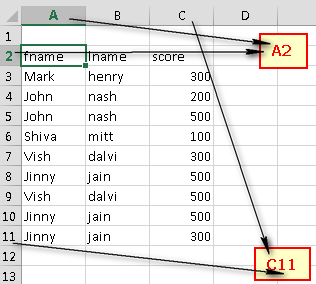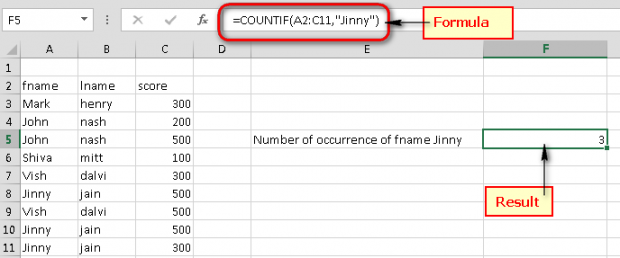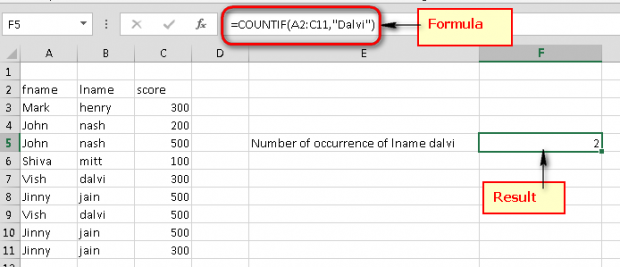# Microsoft Excel: Count the Number of Occurrences of a Text or Number

Posted May 31, 2013 by Vishwanath Dalvi in Microsoft Excel

If you wish to determine how many times a text or number has been repeated within a range or an entire workbook of Excel, this tech-recipe will guide you through the steps with examples.

We will start with an Excel sheet with the following records between the range of A2 to C11.Using the formula listed below, we can determine the number of occurrences of a text or number or how many times a certain text or number has been repeated within an entire excel sheet.

This formula will find the text or number given as criteria and count all the results containing the search term (criteria) within a specified range.

``=COUNTIF(Range, Criteria)``

### Example 1.1

Find the number of occurrences of the first name “Jinny” within a specified range.

``=COUNTIF(A2:C11,"Jinny")``
``Result: 3``### Example 1.2

Find the number of occurrences of the last name “Dalvi” within a specified range.

``=COUNTIF(A2:C11,"Dalvi")``
``Result: 2``### Example 1.3

Find the number of occurrences of the score 500 within a specified range.

``=COUNTIF(A2:C11,500)``
``Result: 4``I am sure this will help you while working with Excel. If you find more ways to count the number of occurrences of an item, please feel free to post them in the comments below.